Memorizing math facts is a skill that is beneficial to young learners and one they'll continue to use throughout their adult lives. Math is essentially everywhere and it's applicable in many fields; therefore, memorizing math facts is a skill students will continue to use regardless of their vocation in the future.

Few students learn basic multiplication concepts in the second grade, but they are expected to master the multiplication tables in their third grade, when they are generally between 7 and 9 years of age.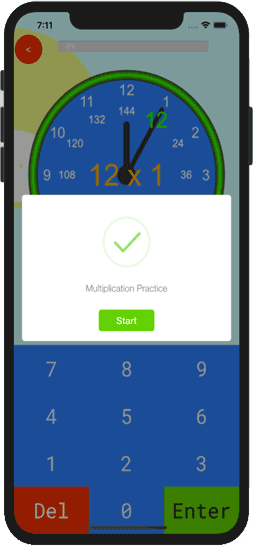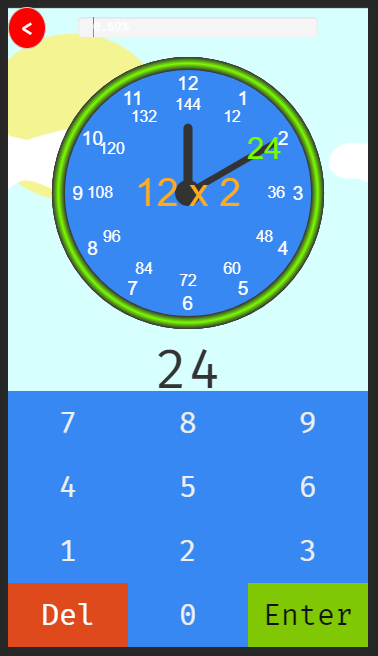Although students are expected to master multiplication facts in their third grade, students in the lower grades can still learn these concepts at home with the help of their parents.

While there are various memorization techniques for learning math facts, the learning style of the student needs to be considered during the learning process. For instance, an auditory learner will have some difficulties learning multiplication facts if you simply wrote the multiplication facts on flash cards and asked them to memorize by visualization.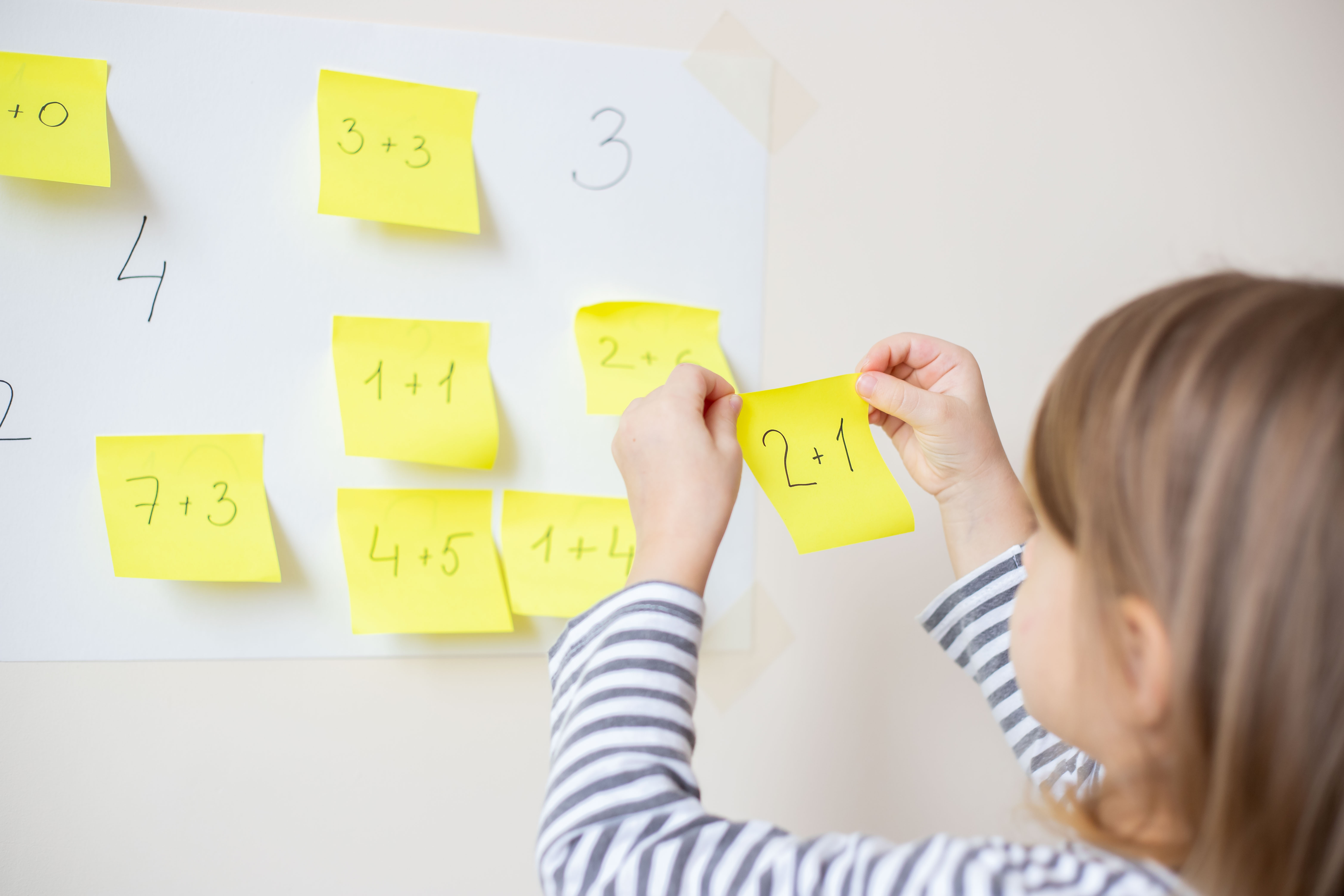Before addressing ways to memorize multiplication facts, we will address some related questions, which will provide some context to the recommended Sqooasha Multiplication Clock (Sq-Mc) memorization method. The questions that will be addressed include:

Although it is recommended that you go through this entire article, you may skip down to the section that specifically addresses the memorization of multiplication facts.

## When should a child memorize multiplication?

Multiplication concepts are usually covered in the third grade after students have already learned basic addition and subtraction.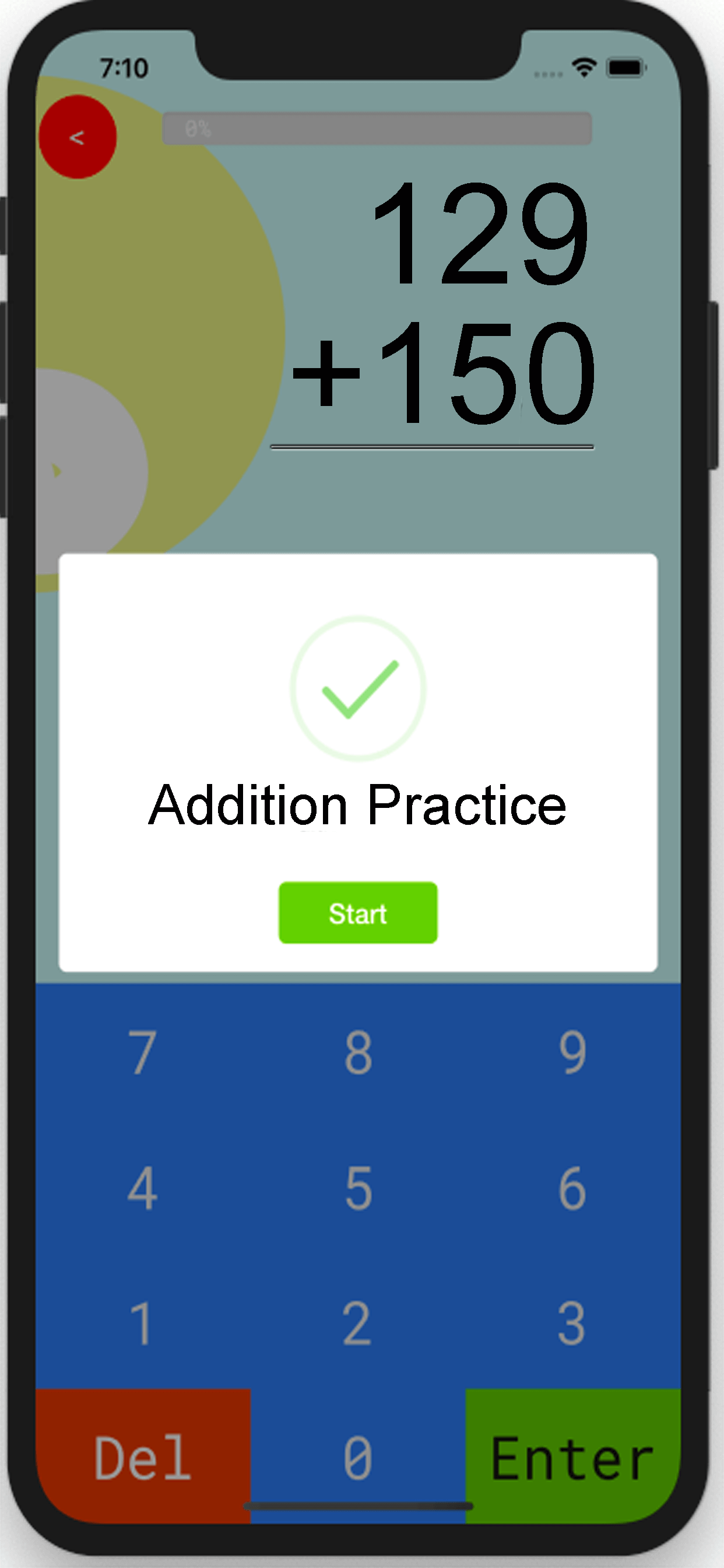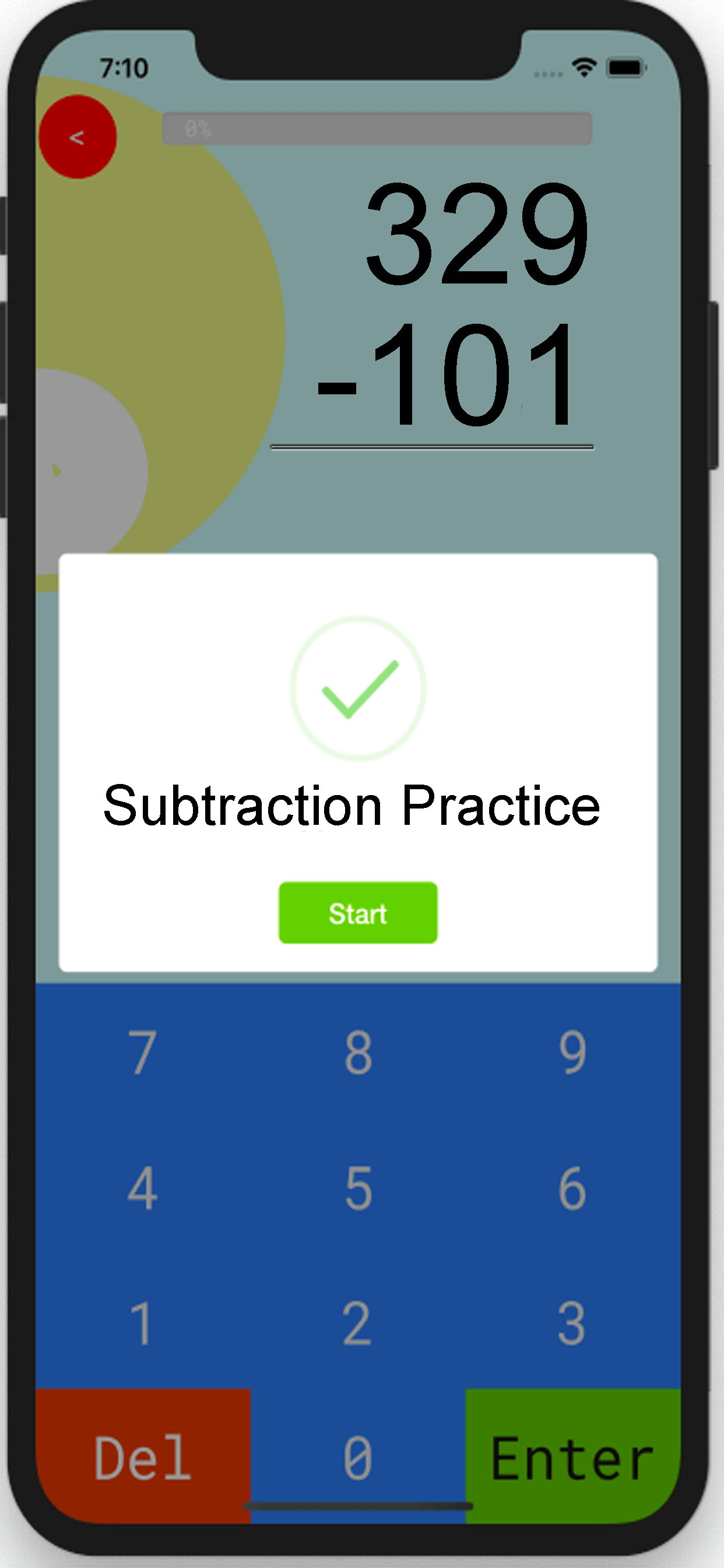Basic math practice on subtraction.

However, memorizing the entire multiplication table all at once, that is, multiplication table 1 through multiplication table 12, can be overwhelming even for a third grader.

This memorization can be less overwhelming if the learning process is divided into manageable scopes and spread over time depending on the learning style and pace of the student.

## How can students remember multiplication facts?

A common question parents ask is "How can I help my child memorize math facts?"

Depending on the learning style of the student, there are a number of ways to help students know the multiplication tables by heart. Some of the commonly used methods include:

• Math-drills
• Rote memorization
• Memorization using songs
• Memorization using games

With all these available options, the main question is "where does a parent start?" Deciding which option to begin with is not an easy task for most parents, especially if a parent is unsure of the learning style of the student. Moreover, the parents may not even know the appropriate steps to follow for any one option.

Furthermore, going through each of the commonly used methods with any one student can be overwhelming for the parent, never mind the student.

## What is the fastest way to memorize multiplication facts?

So far, the importance of using a memorization technique that is specific to the learning style of the student has been underscored.

Since parents may not know the learning style of their children, the method we'll discuss involves a combination of techniques that is applicable to students that are visual, auditory, kinesthetic and/or verbal learners.

Sqooasha offers an automated version of the Sq-Mc method, which uses an item that most students are familiar with: a clock. The step-by-step process of this method will be outlined.

This step-by-step process is outlined to give parents the option of taking the do-it-yourself (DIY) route, with the flexibility of modifying the outlined steps where appropriate, which can be a fun activity for students and their parents.

Although it's helpful for students to know the basics of multiplication before starting the memorization process, it is not a mandatory requirement for this Sq-Mc method, as the parent can provide the additional instructions as needed.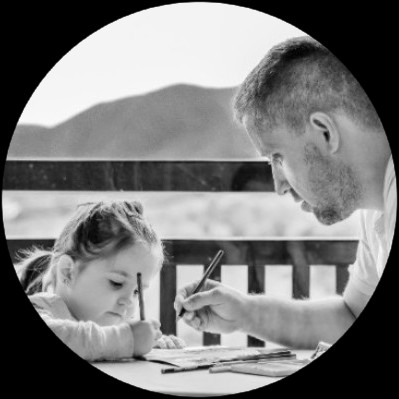The Sq-Mc method can be broken into the following sections:

• 1.
Breaking the memorization scope into small groups of number pairs.
• 2.
Memorizing the answers to the small groups of paired numbers by typing in the answers (kinesthetic), hearing the answers (auditory), saying the answers (verbal) and observing the displayed answers (visual).
• 3.
Practicing with the number pairs until the student recalls the answers with ease.
• 4.
Introducing a new set of number pairs and repeating steps 2 and 3.
• 5.
Combining steps 2, 3, and 4 to practice the combined set of number pairs that have been covered so far.
• 6.
Introducing a new set of number pairs while gradually expanding the scope of practice until the entire multiplication table is mastered.

A key component of the Sq-Mc method is the encouraging words that are provided to students while memorizing the multiplication facts.

The objective of these words of encouragement is to help boost the student's confidence while committing these multiplication facts to memory. The above steps are better explained with an example.

Suppose a learner wishes to memorize the 3 times table - 3 x 1 through 3 x 12 - using the Sq-Mc method, the subsequent sections explain how this learner would proceed to commit this times table to memory.

### Break the multiplication facts into small sets

In the Sq-Mc method, the multiplication facts are divided into paired groups of {1,2}, {3,4}, {5,6}, {7,8}, {9,10} and {11,12}.

The answer to each pair of numbers, starting with the first pair, that is 3 x 1 and 3 x 2, will be memorized and practiced until the student can say/enter the answers with ease before moving to the next pair.

In addition to seeing, hearing, and entering the answers for each pair, the student is encouraged to verbally say the answers as the student may find this method to be their learning style.

The next sections present the detailed example starting with the first number pair.

A high-level overview of this method is presented in a video format. Check out the Sq-Mc summary video below.

### Memorize each number pair

Starting with the first number pair, 3 x 1 and 3 x 2, the Sq-Mc method initially shows the answers to the multiplication facts. All the student has to do is enter the answer as shown using a keyboard, or simply say the answer if you choose to take the DIY approach.

In this example, the multiplication fact questions are in the center of the clock and in orange, while the answers are highlighted in bright green. The student is encouraged to use their favorite colors, which may be particularly useful to visual learners.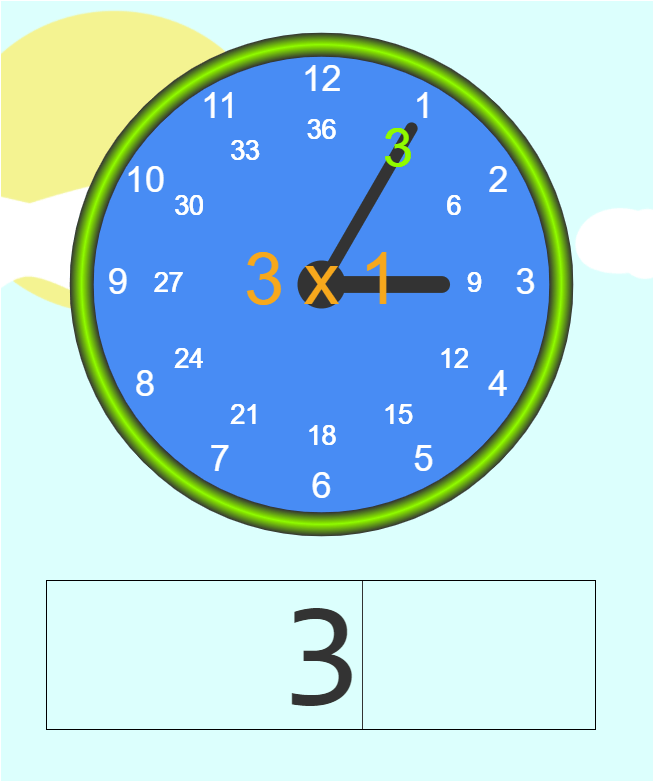First multiplication fact question with answer shown. Student enters three as the answer to 3 x 1.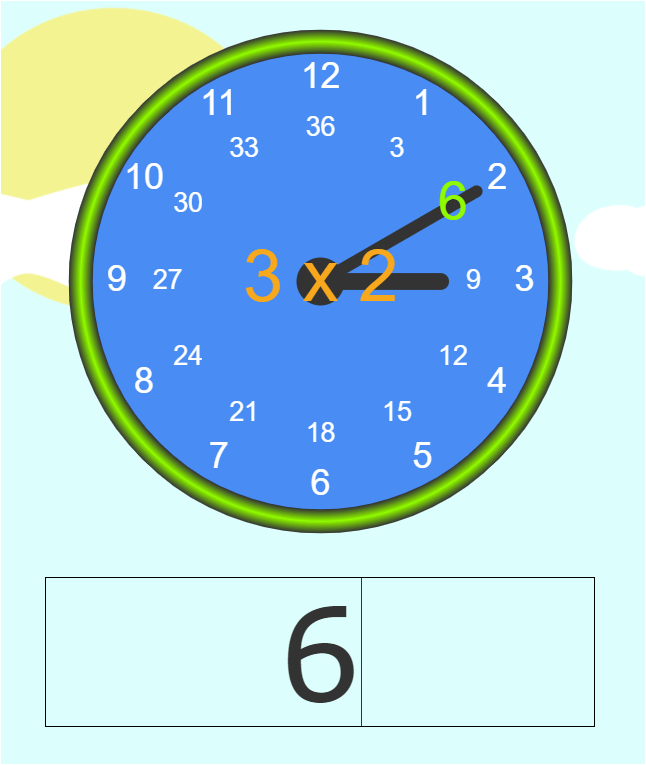Second multiplication fact question with answer shown. Student enters six as the answer to 3 x 2.

It is advisable to inform students that the answers will later be hidden from them, after which they'll have to enter the answers by themselves. This helps the students know what to expect and encourages them to pay closer attention to the initially displayed answers.

### Practice until the student recalls with ease

After showing the first pair of multiplication fact by alternating between the two numbers a few times, hide the answers and have the student enter the answers by themselves.

For instance, using the first number pair, the following questions would be displayed to the student but without the answers.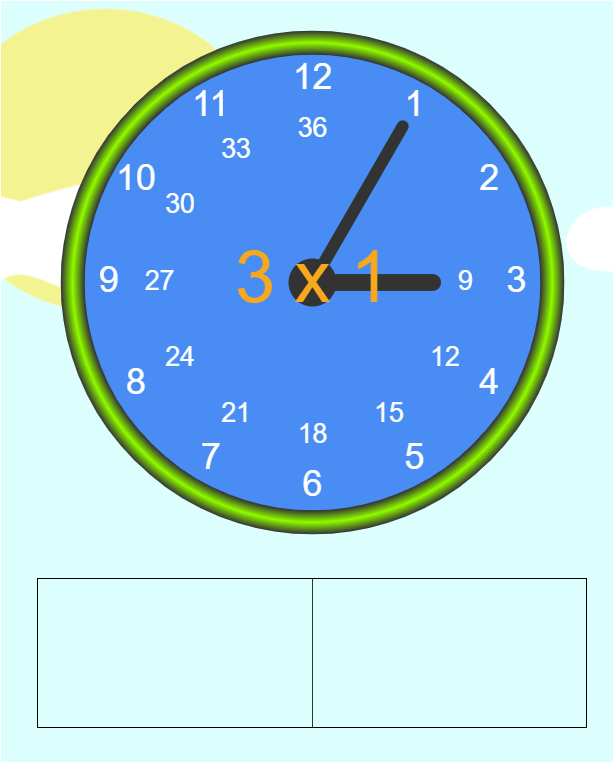Multiplication fact 1 question for the student to practice.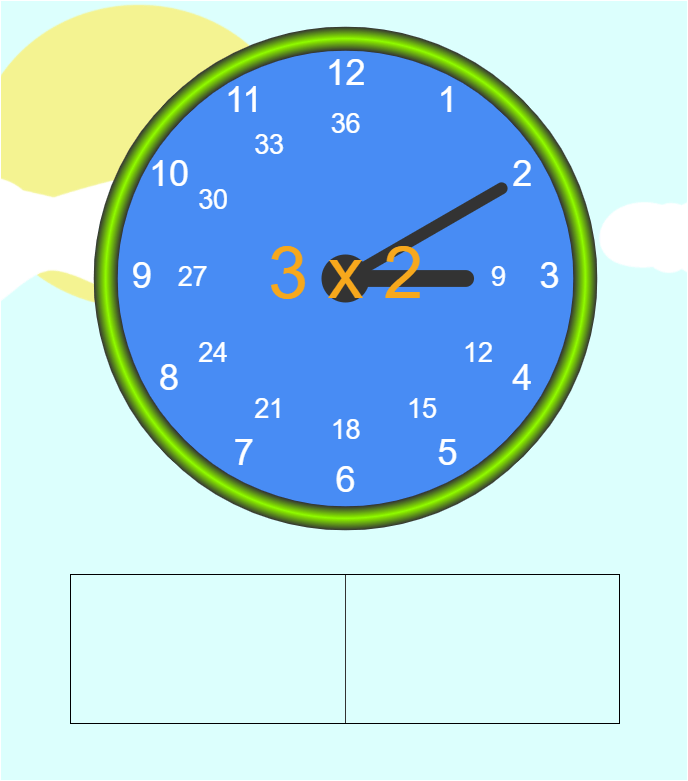Multiplication fact 2 question for the student to practice.

With the answers hidden from the student, they would have to enter the answer to the questions based on what they recall from the initially displayed answers.

The student is ready to move to the next pair of multiplication facts if they can answer the questions with ease, which could be roughly three to five seconds after the multiplication question is presented.

The response time to answer a multiplication question may be slightly different from student to student; however, consistent practice after going through this exercise will help to improve the student's recall and automaticity.

### Add a new number pair to the practice set

After the student demonstrates that they can answer the first set of multiplication facts with ease, the next question pair, which includes 3 x 3 and 3 x 4, are presented to the student as was done for the initial pair of numbers.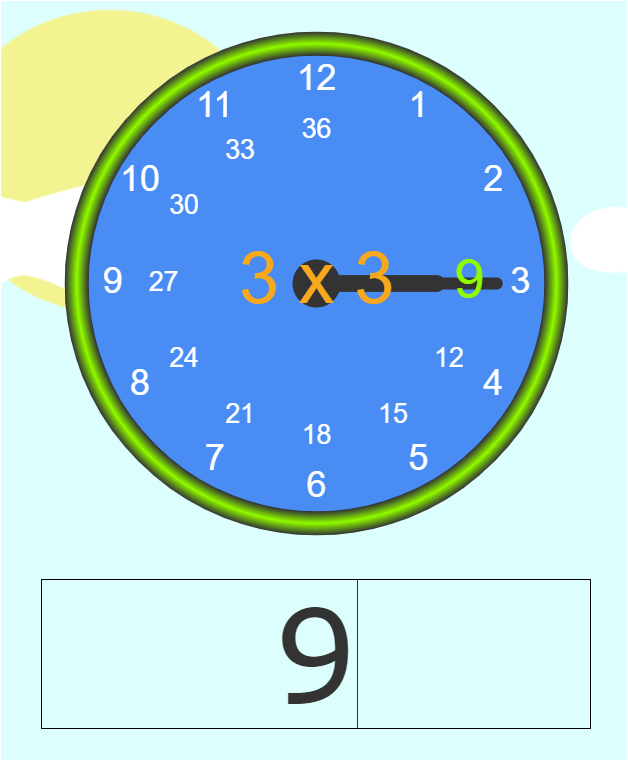Multiplication fact 3 question for the student to practice.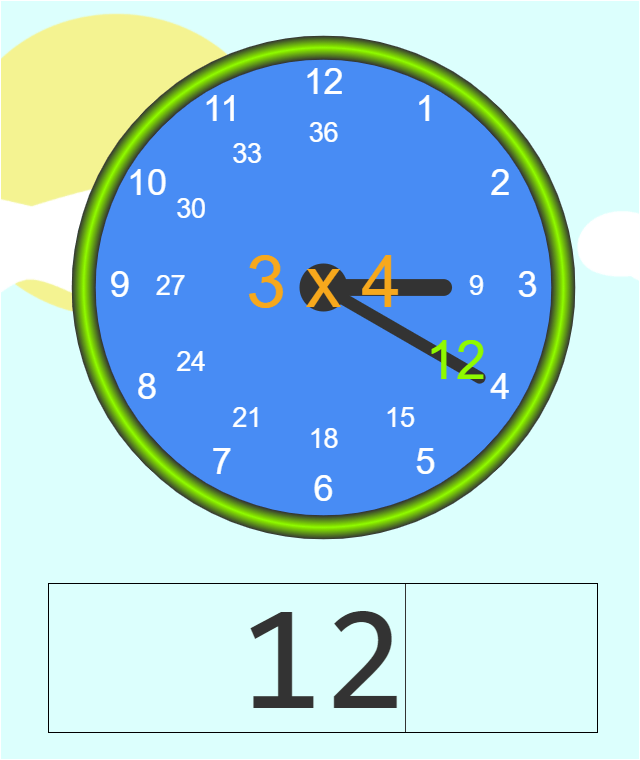Multiplication fact 4 question for the student to practice.

After practicing with the answers displayed, the answers are hidden from the student who now has to try to answer the questions on their own.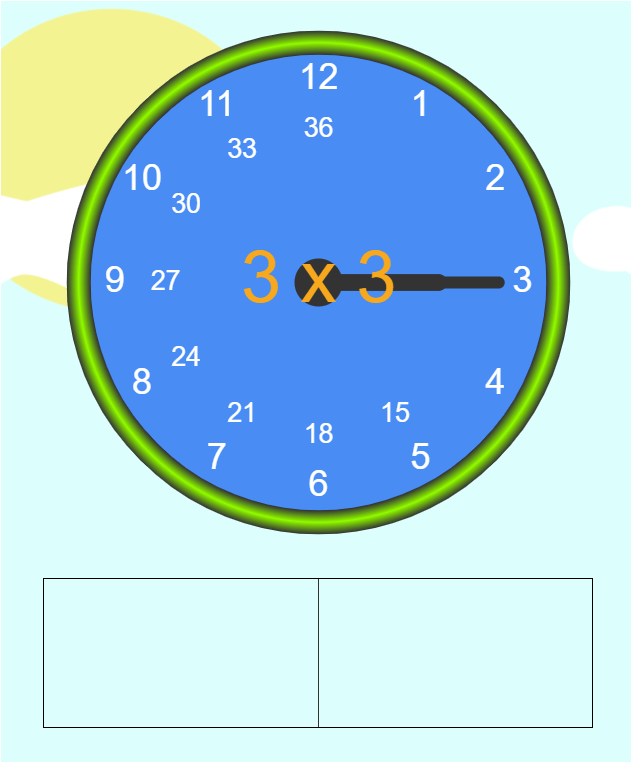Quiz on multiplication fact 3 for the student to practice and memorize.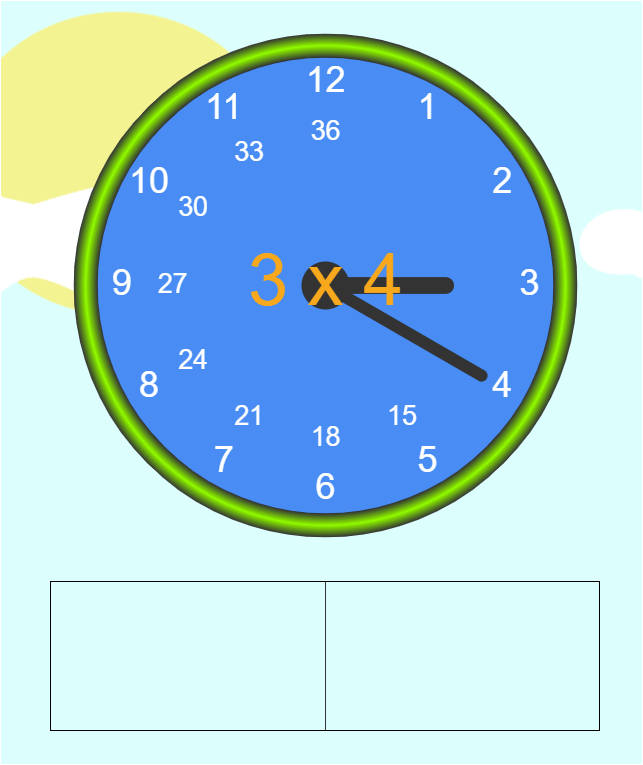Quiz on multiplication fact 4 for the student to practice and memorize.

### Combine previously learned multiplication facts and practice as a set

Upon mastering the second pair of multiplication facts, it is recommended that the student goes over the combined set of previously learned multiplication facts before moving onto the next pair.

In this example, the student would go over 3 x 1, 3 x 2, 3 x 3 and 3 x 4, with all the answers hidden from the student. In other words, the student will have to enter the answers by themselves.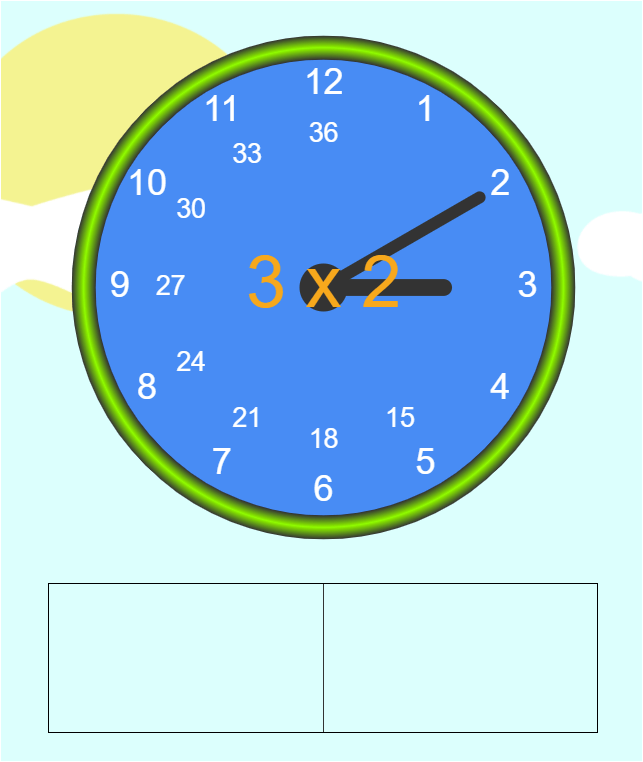Quiz on all the multiplication facts covered so far, which includes facts 1 through 4.

Although you can present the already covered multiplication facts in any desired order, it is much more spontaneous and fun to randomize the order this combined set of number pairs are present.

### Gradually expand the number set until the entire multiplication table is mastered

The foregoing steps cover the basis from which the remaining multiplication facts are memorized. These remaining multiplication facts are shown pictorially in this section.

As the set of multiplication facts to memorize increases, it is normal for the student to occasionally forget some of the previously covered multiplication facts.

This is a situation where learning the basics of multiplication before memorizing the facts can be invaluable. From the knowledge of the basics, the student can attempt to derive the answer to the forgotten multiplication fact while working to commit the answer to memory.

If the student is unable to remember a previously covered multiplication fact, provide hints to guide the student to the correct answer. For example, if the student does not remember the answer to 3 x 2, you can remind them that this problem is equivalent to adding 3 two times.

If the student has already learned the two times table, you can remind the student that 3 x 2 is the same as 2 x 3, and show the student why these two questions are similar.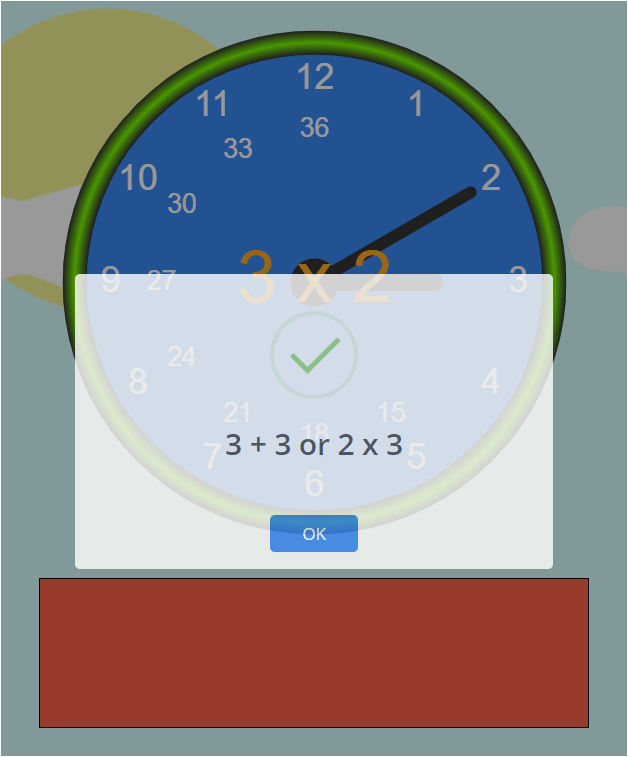Hint for multiplication fact the student was unable to answer.

Providing words of encouragement while the student practices to commit the multiplication facts to memory can go a long way to boost their overall mathematical confidence.

Therefore, providing these words of encouragements as the student enters the correct answer is highly recommended.

Following the outlined sequence, the remaining multiplication facts are memorized in the predefined order as follows:

Practice the next multiplication set, which includes 3 x 5 and 3 x 6.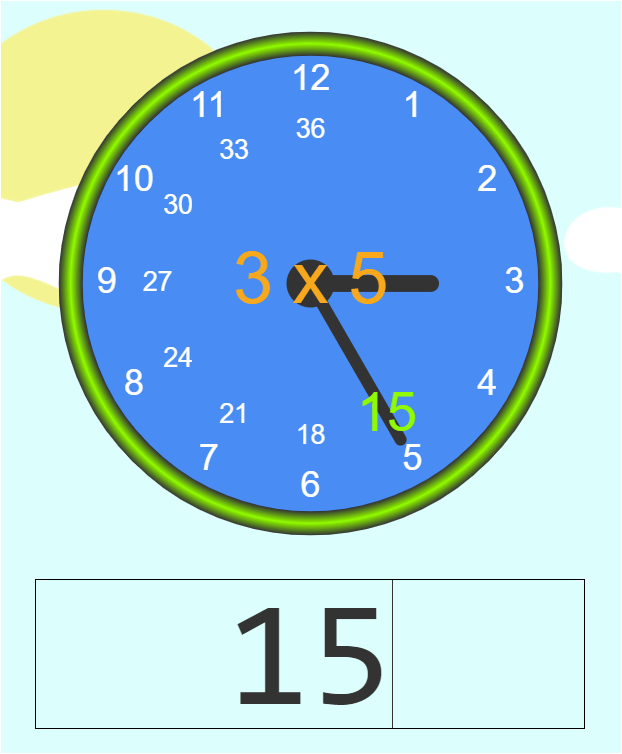Multiplication fact 5 for the student to practice.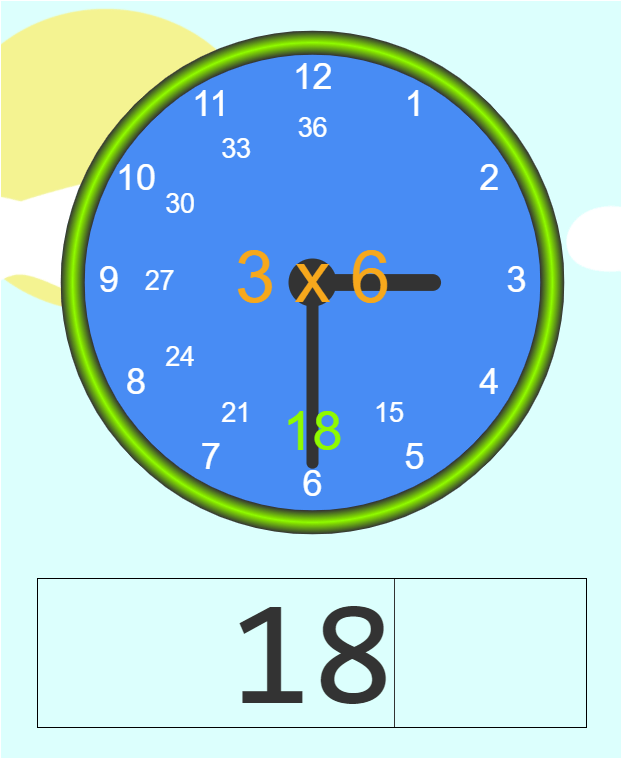Multiplication fact 6 for the student to practice.

Take a quiz on this new multiplication set of 3 x 5 and 3 x 6.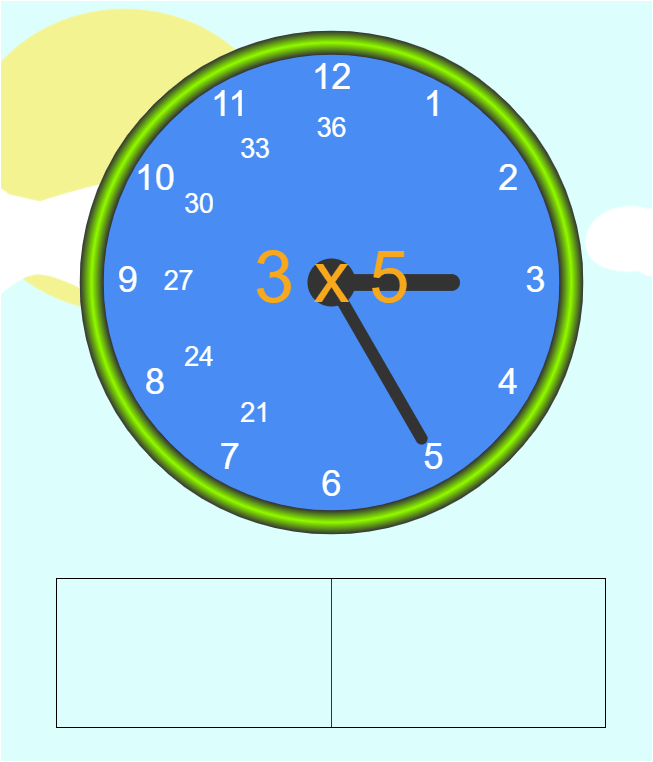Quiz on multiplication fact 5 for the student to practice and memorize.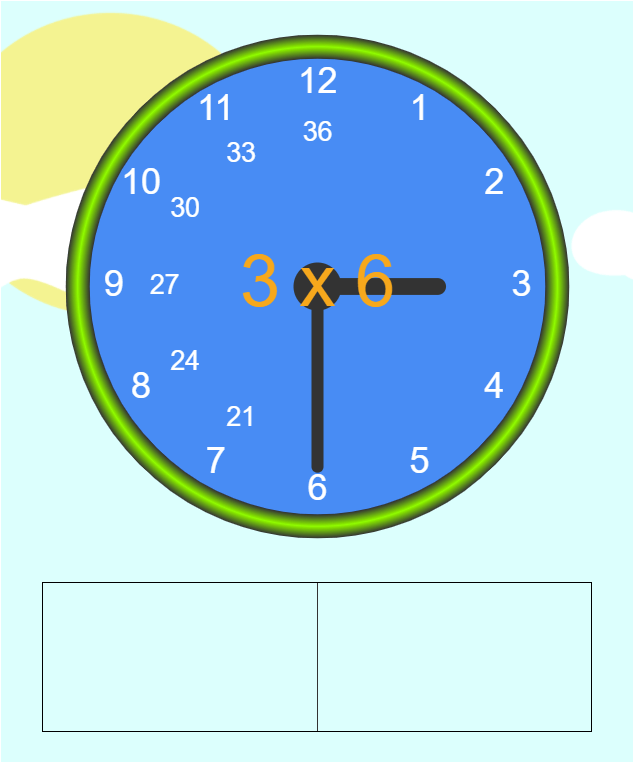Quiz on multiplication fact 6 for the student to practice and memorize.

Take a quiz on a randomized, combined set of 3 x 1, 3 x 2, 3 x 3, 3 x 4, 3 x 5 and 3 x 6.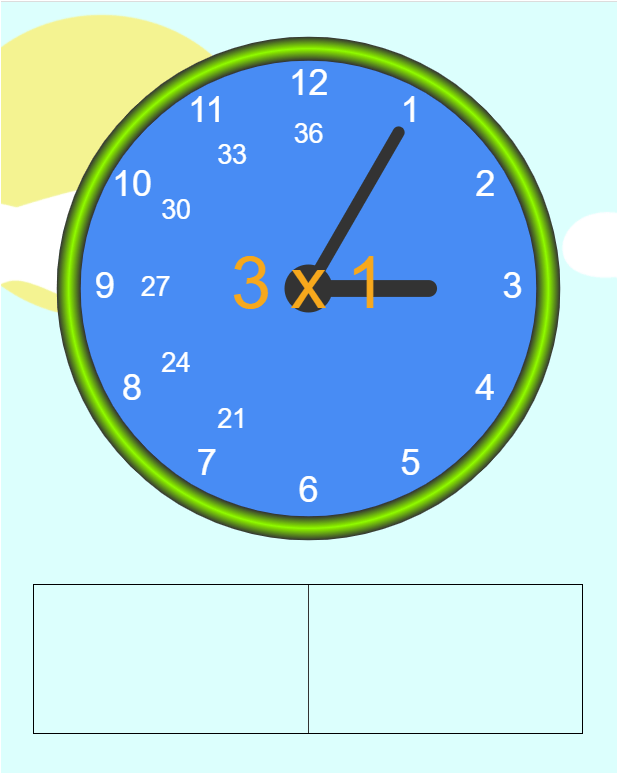Quiz on all the multiplication facts covered so far, which includes facts 1 through 6.

Practice the next multiplication set, which includes 3 x 7 and 3 x 8.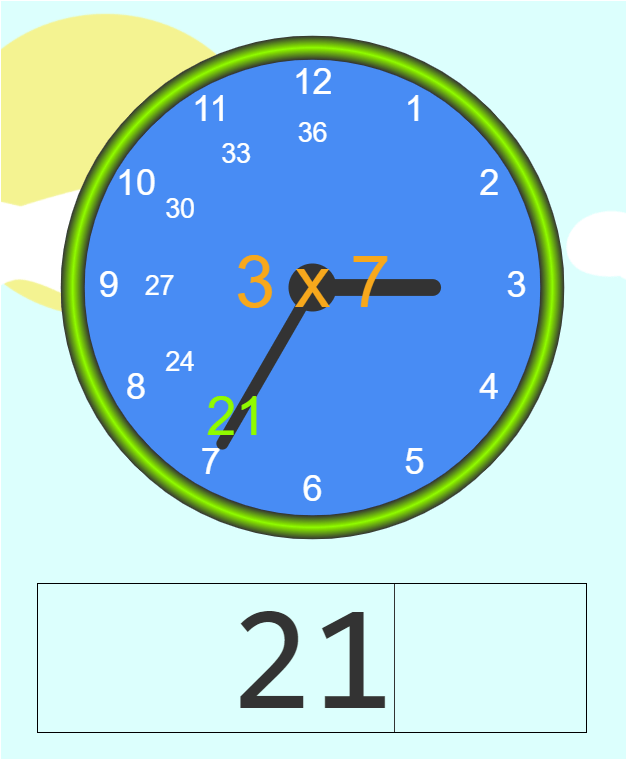Multiplication fact 7 for the student to practice.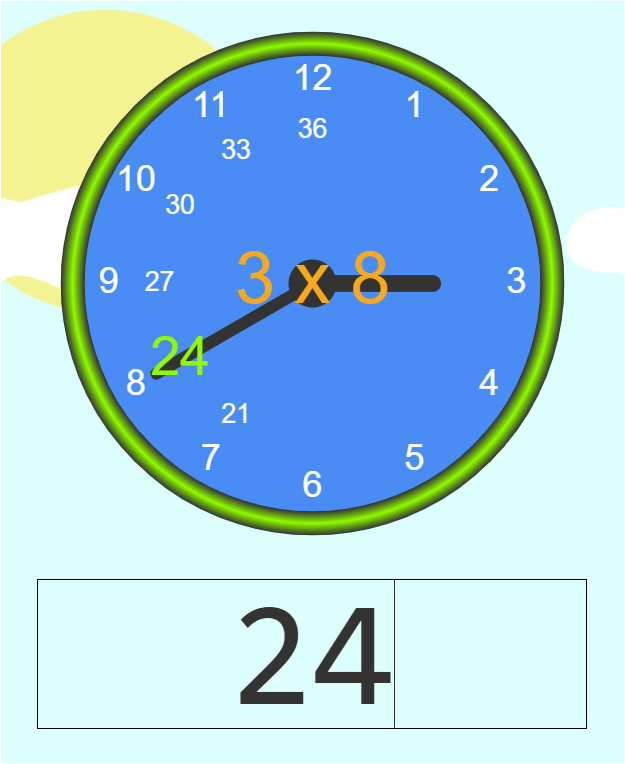Multiplication fact 8 for the student to practice.

Take a quiz on this new multiplication set of 3 x 7 and 3 x 8.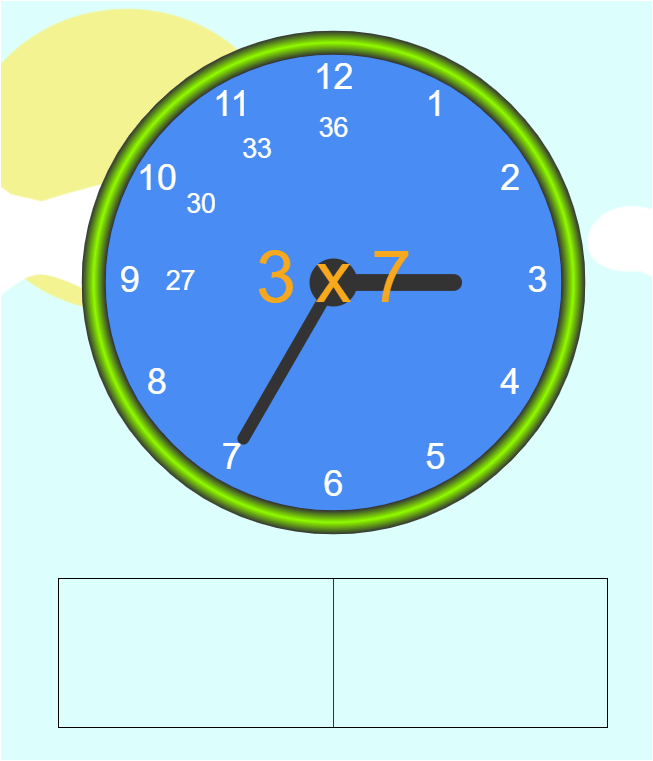Quiz on multiplication fact 7 for the student to practice and memorize.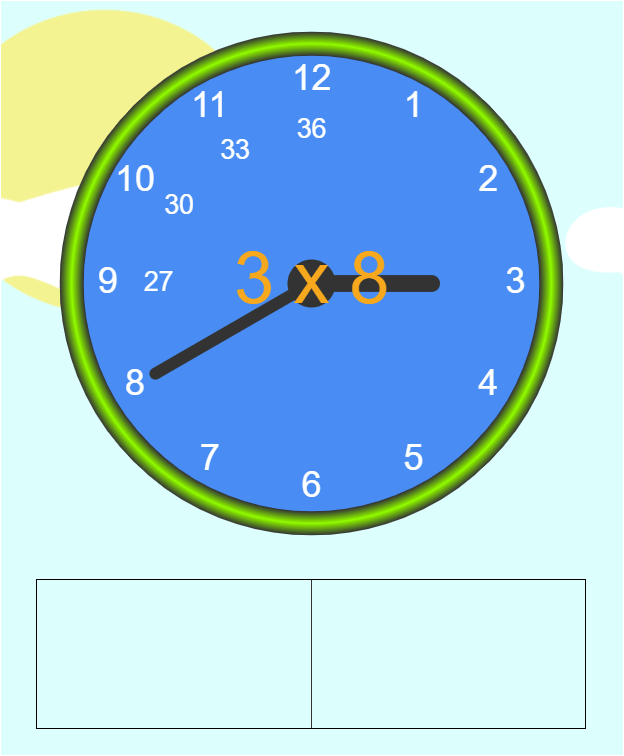Quiz on multiplication fact 8 for the student to practice and memorize.

Take a quiz on a randomized, combined set of 3 x 1, 3 x 2, 3 x 3, 3 x 4, 3 x 5, 3 x 6, 3 x 7 and 3 x 8.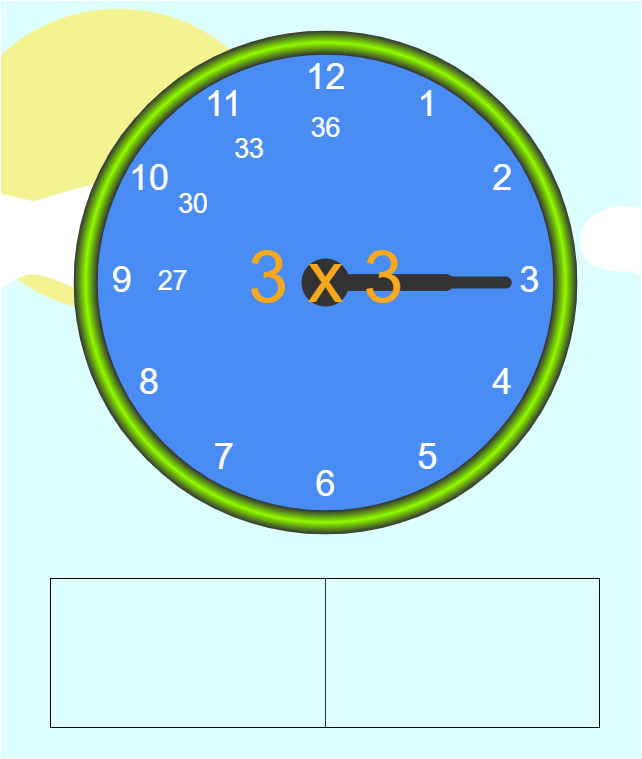Quiz on all the multiplication facts covered so far, which includes facts 1 through 8.

Practice the next multiplication set, which includes 3 x 9 and 3 x 10.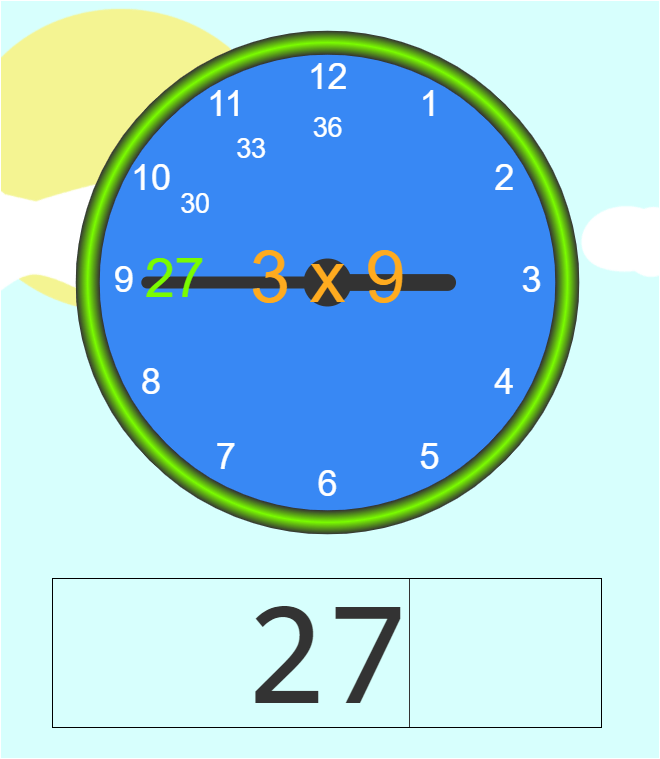Multiplication fact 9 for the student to practice.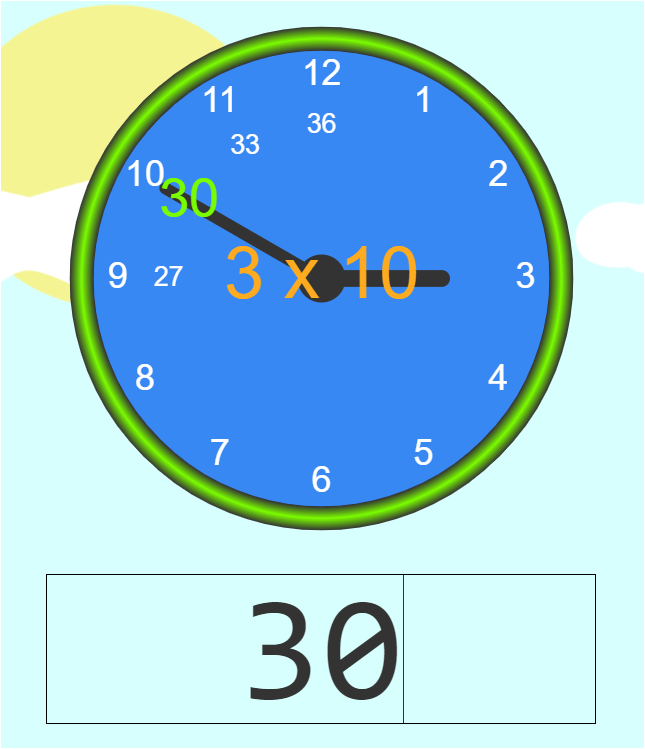Multiplication fact 10 for the student to practice.

Take a quiz on this new multiplication set of 3 x 9 and 3 x 10.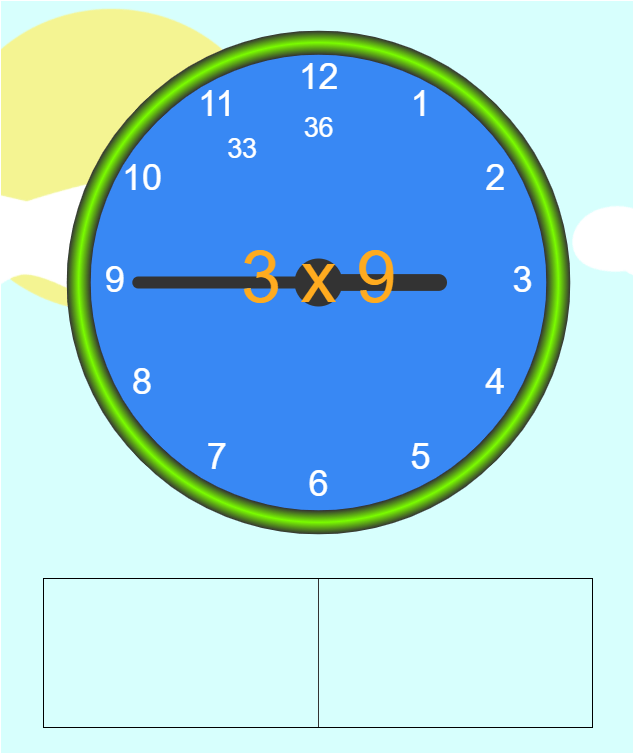Quiz on multiplication fact 9 for the student to practice and memorize.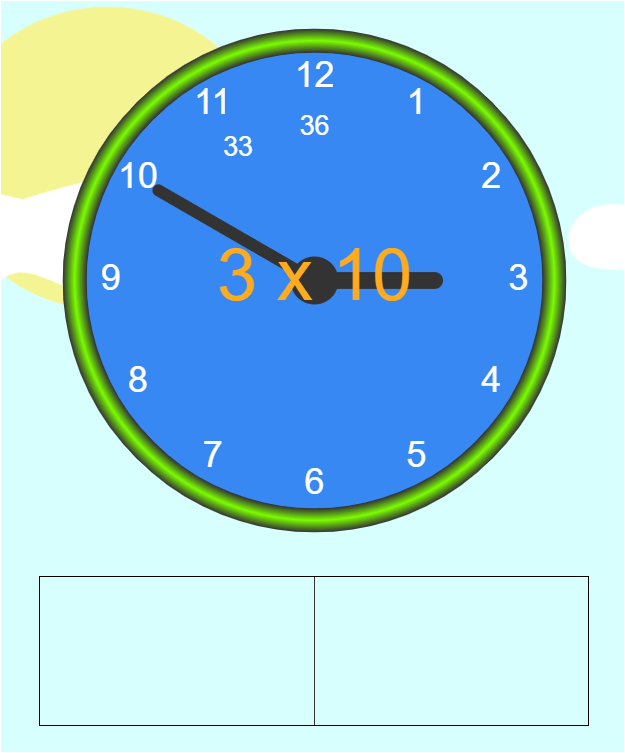Quiz on multiplication fact 10 for the student to practice and memorize.

Take a quiz on a randomized, combined set of 3 x 1, 3 x 2, 3 x 3, 3 x 4, 3 x 5, 3 x 6, 3 x 7, 3 x 8, 3 x 9 and 3 x 10.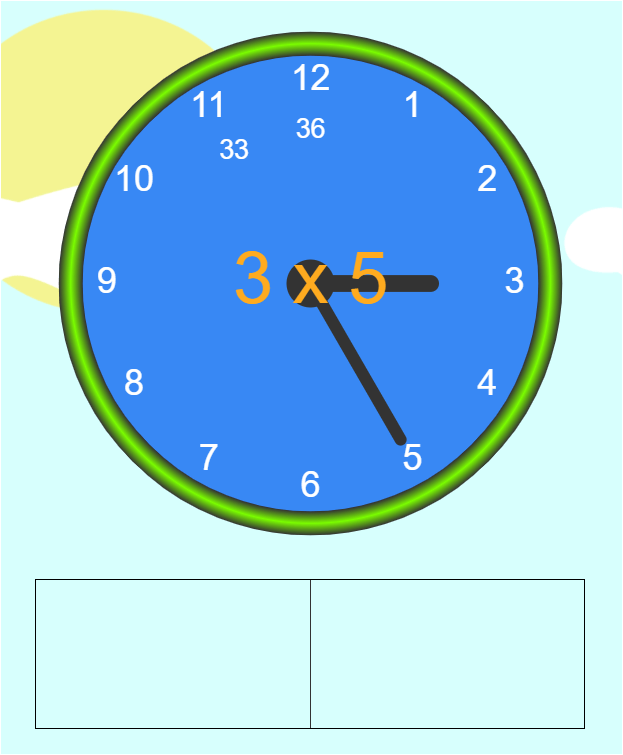Quiz on all the multiplication facts covered so far, which includes facts 1 through 10.

Practice the next multiplication set, which includes 3 x 11 and 3 x 12.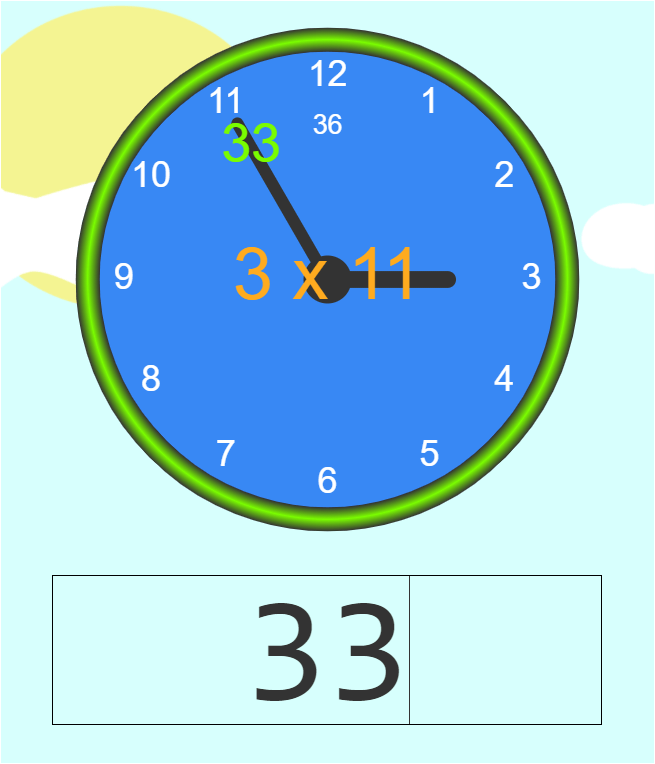Multiplication fact 11 for the student to practice.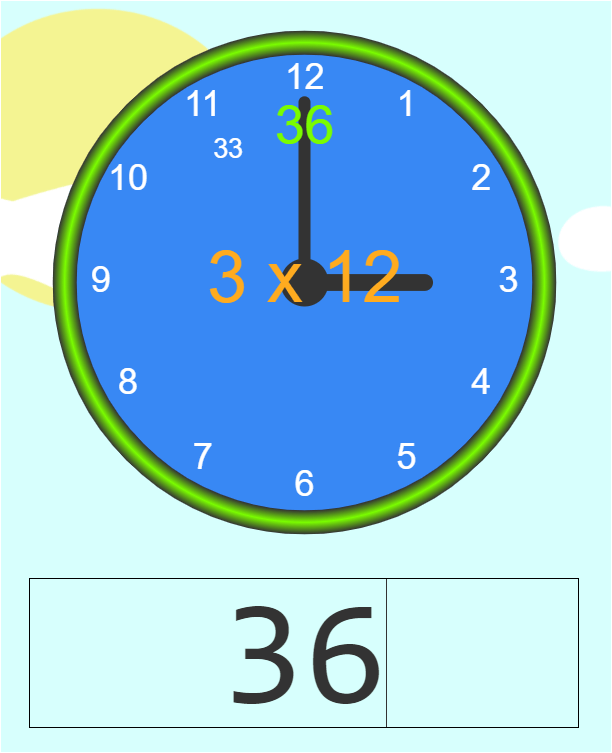Multiplication fact 12 for the student to practice.

Take a quiz on this new multiplication set of 3 x 11 and 3 x 12.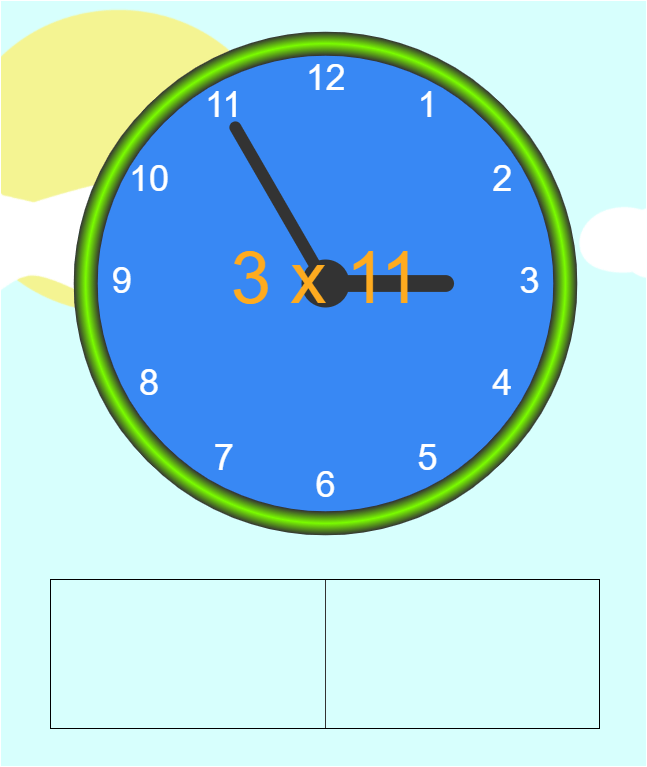Quiz on multiplication fact 11 for the student to practice and memorize.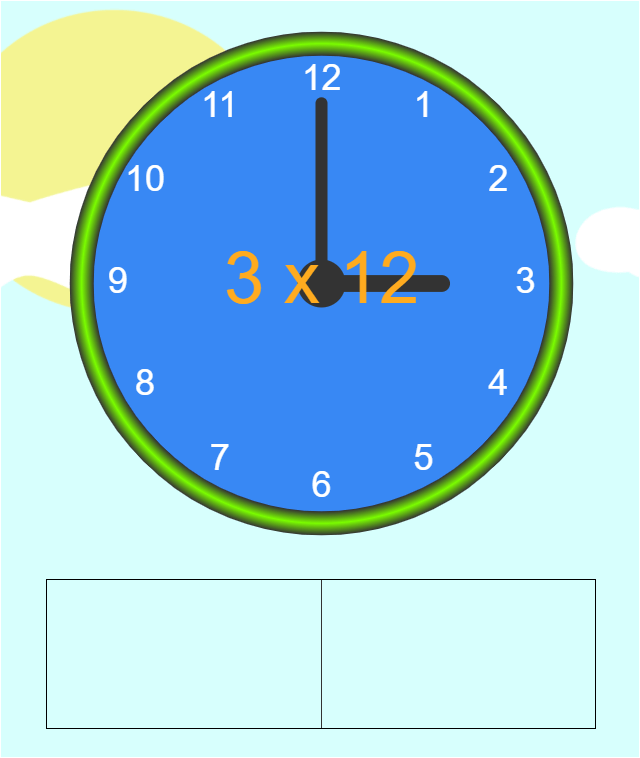Quiz on multiplication fact 12 for the student to practice and memorize.

Take a quiz on a randomized, combined set of 3 x 1, 3 x 2, 3 x 3, 3 x 4, 3 x 5, 3 x 6, 3 x 7, 3 x 8, 3 x 9, 3 x 10, 3 x 11 and 3 x 12.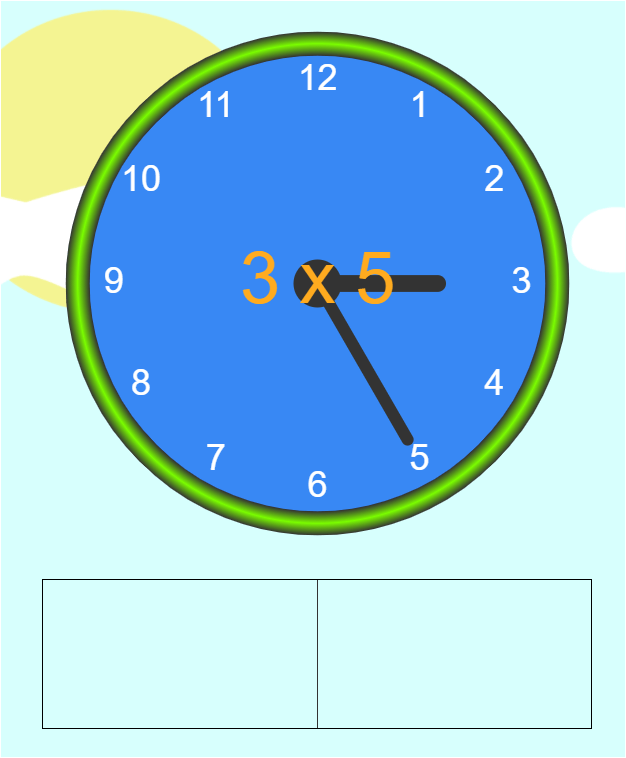Quiz on all the multiplication facts covered so far, which includes facts 1 through 12.

## Next steps to solidify the memorized multiplication facts

Congratulations, you have just learned the Sq-Mc method and are ready to implement it with your students. Although this illustration focused on the 3 times table, the same concepts can be applied to any multiplication table.

To quickly get you started, here are some multiplication worksheets, which complement this example.

You may also want to check out this useful tool that provides the solutions to multiplications facts in multiple choice format.

After learning a given times table, it is important for the student to continue practicing to improve his overall response time.

If you are considering the DIY method for your child to start his/her multiplication practice, designing a tool that implements the Sq-Mc method can be an involved task. Sqooasha already has a system that automates this process, including the randomization of the practice questions and audio praises to encourage your student along the way.